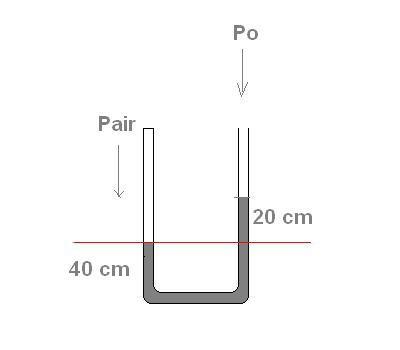# U-Tube (Pv=nRT, Pascals) Problem

Challenge problem

1. Homework Statement

A U-tube of uniform cross section contains mercury. The initial temperature of the system is 301K and the barometric pressure is 749 torr. Suppose the left side of the tube is closed at the top and the temperature is raised to a temperature such that the air column on the left is 60cm high. Determine the temperature at which this occurs.

Initially, the mercury level is 50 cm on both sides. The height of each tube, as measured from the bottom, is 100 cm.

## Homework Equations

Ideal gas law PV=nRT

Pascals principle P = P0+ρgh

## The Attempt at a Solution

I know what my initial pressure and temperature is. In addition I can write my initial volume on the left tube as A(.50) .

My goal is to find the temperature when the the column of mercury on the left tube drops by 10cm (air column now 60cm).

So now I want to develop an expression to describe the final state of the left side of the tube.

Im assuming the number of moles will not change & R is a constant of course.

PliVli/(Ti) = PlfVlf/(Tf)

So now I have one equation and two unknowns ( I dont know the final pressure or temperature, the volume can be written in terms of AH). So I need to develop another expression. Here is where I can take full advantage of pascals principle.

When the mercury goes down on the left side & goes up on the right side, the pressure on the left side of the tube, at the "surface" of the mercury, must be equal to the pressure on the right tube at the same point.

The pressure on the right side of the tube can be written as

P = Pairhggh

Where, h= 0.20m . I choose 0.20m because when the left side goes down by .10m the right side goes up by .10m. Thus if I want to look at the pressure on the right side, I must acknowledge that the point is 0.20m below the surface of the liquid.

So now I can say Plf = P = Pairhggh

∴ I am now ready to solve for Tf .

Issue is, when I went to solve for Tf , I got an answer that did not correspond to one of the solutions.

here are the values I used

ρhg = 13500 kg/m^3
h = 0.20m
Pair = 101325 pa
Pli = 749 torr = 99858 pa
Vli[/SUB = A(0.50m)
Vlf[/SUB = A(0.40m)

And of course the initial temperature is 301 K.

Bystander
Homework Helper
Gold Member
I went to solve for Tf , I got an answer

PliVli/(Ti) =PlfVlf/(Tf)

Tf =PlfVlfTi /(PliVli)

Note:
Plf = Pair + ρhggh = (101325)+(13500)(9.80)(.20) = 127785 pa

Subbing in values:

Tf = (127785) (A(0.40))(301) / ((99858)(A(0.50)) = 308 K

ehild
Homework Helper
What is the final volume of the gas on the left?

What is the final volume of the gas on the left?

I argue that the final volume on the left side will be, Vlf = A(0.40) .

The 0.40 is the height of the fluid on the left.

Is this a correct statement?

ehild
Homework Helper
Why did you use the height of the fluid when the gas law applies to gases?????

Bystander
Homework Helper
Gold Member
initial temperature of the system is 301K and the barometric pressure is 749 torr

Why did you use the height of the fluid when the gas law applies to gases?????

Goodness me, I have no idea.

I now used (0.60) instead of (0.40) , I managed to get about 460K now.

I dont follow, is the pressure given not an absolute pressure?

Bystander
Homework Helper
Gold Member
I dont follow
Yes you do.
Pli = 749 torr = 99858 pa

Yes you do.

749 torr =/= barometric 749 torr ?

ehild
Homework Helper
You can measure the pressure in torr-s - the height in mm of a mercury column of equivalent pressure. So the 20 cm mercury column means additional 200 torr pressure. No need to convert to pascals.
The density of mercury depends on temperature, and so does its length, but assuming that only the air column is heated you can ignore it.

You can measure the pressure in torr-s - the height in mm of a mercury column of equivalent pressure. So the 20 cm mercury column means additional 200 torr pressure. No need to convert to pascals.
The density of mercury depends on temperature, and so does its length, but assuming that only the air column is heated you can ignore it.

This is quite new to me, so excuse me if I sound a bit ignorant, but

1 cm of mercury column constitutes 10 torr pressure?

Therefore when I consider the 20cm mercury column, I should add 200 torr pressure. Consequently, I should account for the 40cm mercury column on the left tube?

ehild
Homework Helper
The 40 cm column in the left tube is below the air column, it does not add to the pressure. See attachment. The downward force at the red level has to be equal in both tubes, so the air pressure in the left tube is equal to the atmospheric pressure+ the pressure of the 20 cm mercury column.•AKJ1
The 40 cm column in the left tube is below the air column, it does not add to the pressure. See attachment. The downward force at the red level has to be equal in both tubes, so the air pressure in the left tube is equal to the atmospheric pressure+ the pressure of the 20 cm mercury column.

View attachment 78923

Very clear explanation. Got it, thank you!

ehild
Homework Helper
You are welcome :).